Courses

# Test: Inventory Control (Level - 3)

## 18 Questions MCQ Test Manufacturing and Industrial Engineering | Test: Inventory Control (Level - 3)

Description
This mock test of Test: Inventory Control (Level - 3) for Mechanical Engineering helps you for every Mechanical Engineering entrance exam. This contains 18 Multiple Choice Questions for Mechanical Engineering Test: Inventory Control (Level - 3) (mcq) to study with solutions a complete question bank. The solved questions answers in this Test: Inventory Control (Level - 3) quiz give you a good mix of easy questions and tough questions. Mechanical Engineering students definitely take this Test: Inventory Control (Level - 3) exercise for a better result in the exam. You can find other Test: Inventory Control (Level - 3) extra questions, long questions & short questions for Mechanical Engineering on EduRev as well by searching above.
*Answer can only contain numeric values
QUESTION: 1

### The following data is given for an inventory control system. The annual demand is given as 24,000 units and the safety stock is 200 units. The economic order quantity is 3000 units. If the lead time is 12 days and the number of working days is 300, then the reorder level is __________ units.

Solution:

Given data,

Annual demand = 24,000 units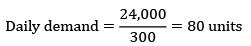safety stock = 200 units

EOQ = 3000 units

LT = 12 days

Working days = 300

ROL = SS + Demand during LT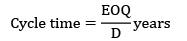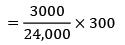cycle time = 37.5

ROL = 200 + 80 × 12

ROL = 1160

*Answer can only contain numeric values
QUESTION: 2

### Consider the following data Annual usage = 12,000 units, carrying cost is given as 10% of the unit cost. The cost of an item is Rs. 100. The ordering cost per order is given as Rs. 60 Due to some technical reasons the supply is restricted to only 350 units. Find the total annual cost.

Solution:

Given,

D = 12,000 units

CC = 10% × 100 = Rs. 10/unit/year

Co = Rs. 60/order

Supply is restricted to 350 units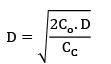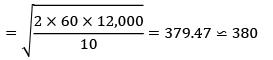EOQ = 380 which is greater than 350 So, find total cost at 350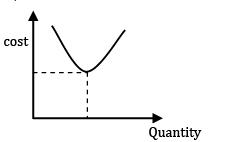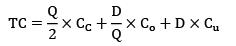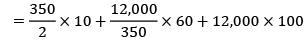= Rs. 12,03,807

QUESTION: 3

### The lead time and consumption pattern of a component is as follows: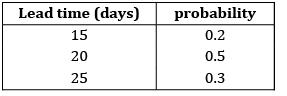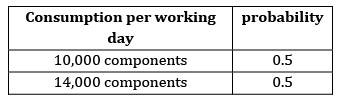If safety stock = 54,000 units. The ROL is

Solution:

= 0.2 × 15 + 0.5 × 20 + 0.3 × 25

= 20.5

Average consumption

= 0.5 × 10,000 + 0.5 × 14,000

= 12,000 units

ROL = SS + Avg. lead time × Avg. consumption

= 54,000 + 20.5 × 12,000

= 3,00,000

*Answer can only contain numeric values
QUESTION: 4

The annual demand for an item is 4000 units, ordering cost is Rs. 200 per order and inventory holding cost is given as 8 Rs./unit/year. If 4% is offered for a lot of 1200 units then what will be economical ordering quantity. (Assume unit cost of an item is Rs. 40)

Solution:

Frequency of production run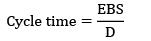Given data,

Production rate = 24,000/day

Consumption rate = 12,000/day

CC = Rs. 0.02/unit/year

Co = Rs. 18/set‐ up

Working days = 300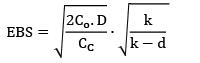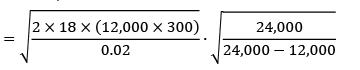= 113841.99

Annual demand = (12,000 × 300) = 36 × 105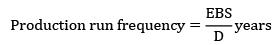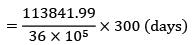= 9.48

≃ 9.5 days

*Answer can only contain numeric values
QUESTION: 5

The daily demand for a commodity is given as 200 units. A fixed cost of Rs.1000 is incurred every time an order is placed. The carrying cost is given as Rs. 0.02 per unit per day. The minimum annual inventory cost is _____________

Solution:

Given data,

Demand = 200 units/day

= 200 × 365 units/year

= 73,000 units/year

Ordering cost = Rs. 1000/order

CC = Rs. 0.02/unit/day

= 0.02 × 365/unit/year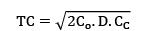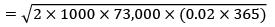TC = Rs. 32646.59 /year

*Answer can only contain numeric values
QUESTION: 6

A particular item has a carrying cost of Rs. 2.4/unit/year. The total variable cost of inventory at EOQ is given as 2160. The annual demand is 9000 units. If the replacement is instantaneous then find-out the optimal number of order/year.

Solution:

Given data,

CC = Rs. 2.4/unit/year

TVC = 2160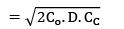Annual demand = 9000 units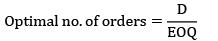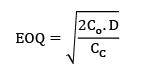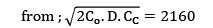2Co. D. CC = 21602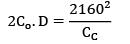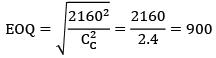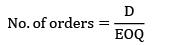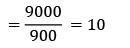QUESTION: 7

For lead time and consumption the probabilities are given in the table below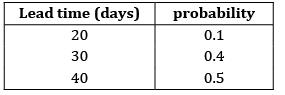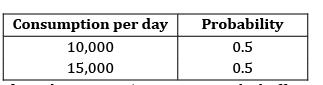If reorder point is 5,00,000 units. The buffer stock is ______________

Solution: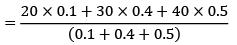= 34 days

Average consumption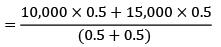= 12500 units ROP or ROL = Buffer stock or safety stock + Avg. LT × avg. consumption

5, 00,000 = SS + 34 × 12500 SS = 75,000 units

QUESTION: 8

A company has an annual demand of 2000 units, ordering cost of Rs. 100/order and a carrying cost of Rs. 200/unit/year. If the shortage costs are estimated to be nearly Rs. 400/unit/year each time the company runs out of stock, then the stock justified by shortage cost is ______________

Solution:

Given data,

D = 2000 units/year

Co = Rs. 100/order

CC = Rs. 200/unit/year

CS = Rs. 400/unit/year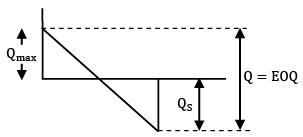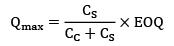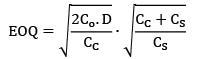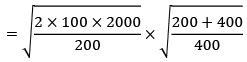= 54.77 units

≃ 55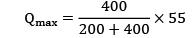Qmax = 36.67 ≈ 37

Qmax + QS = EOQ

QS = 55 − 37

QS = 18 units

Stock justified by shortage cost

= QS = 18 units

∗ Stock justified by the shortage cost = QS

*Answer can only contain numeric values
QUESTION: 9

The demand during lead time varies uniformly between 8000 units and 12,000 units. The unit price is given as Rs. 4 and the selling price is Rs. 6. The unsold item has a salvage value of Rs. 2. Determine the optimal level of inventory to stock.

Solution:

Selling price per unit = Rs. 6

Cost price per unit = Rs. 4

Salvage value = Rs. 2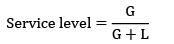Gain = S. P − C. P = 6 − 4 = 2

Loss = C. P − Salvage value = 4 − 2 = 2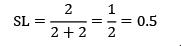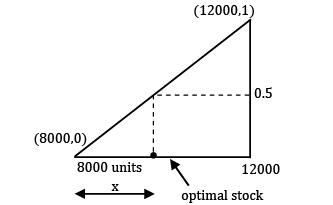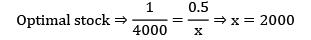∴ Optimal stock = 8000 + 2000 = 10,000

QUESTION: 10

A moulding firm produces and uses 39,000 units annually. The set-up cost is Rs. 20 and production rate per week is 1000 units. If inventory carrying cost is Rs. 15 per unit per annum, the maximum inventory in units is ____________

Solution:

Annual production = 39,000 units

Co = Rs. 20/set‐ up

production rate = Rs. 1000/week

CC = Rs. 15/unit/annum

Qmax =?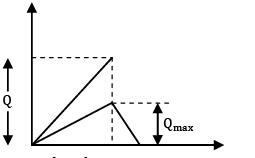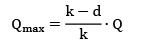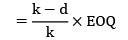k = production rate

= 1000 units/week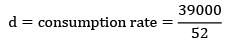= 750 units/week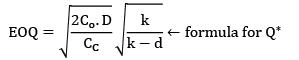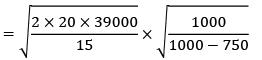= 644.98

≃ 645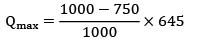Qmax= 161.25 units ≈ 162 units

QUESTION: 11

A company uses 60,000 units per annum of an item, each costing Rs. 120. The ordering cost per order is Rs. 50 and annual inventory carrying costs are 15%. If the company operates 300 days on year, the lead time is given as 10 days and safety stock is 600 units. Find out the re-order level

Solution:

Annual demand = 60,000 units

Unit cost = Rs120/unit

Ordering cost = Rs. 50/order

Carrying costs = 15% × unit cost = Rs. 18/unit‐year

Working days = 300

LT = 10 days

SS = 600 units

ROL = SS + LT × consumption rate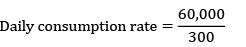= 200 units /day

ROL = 600 + 10 × 200 = 2600 units

*Answer can only contain numeric values
QUESTION: 12

In an industry, two bin system is used to control inventory for a particular component. The first bin hold 1200 units and the second bin holds 800 units. When first bin gets empty second bin is consumed during the lead time. The annual usage of the component is 40,000 units. The cost to place an order is around Rs. 50, the annual holding cost per unit is Rs. 4. The total variable cost for the two-bin approach is __________

Solution: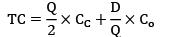In two bin system order is placed after consuming 1st bin So, ordering quantity will be 1200 units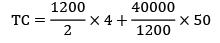= Rs. 4066.67

QUESTION: 13

If the ratio of total incremental cost for a quantity Q to total incremental cost for EOQ quantity is 2, then the ratio of quantity Q to EOQ is _________

Solution: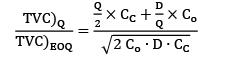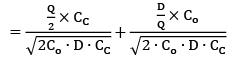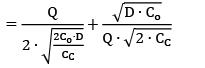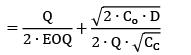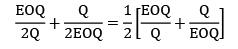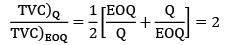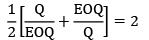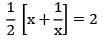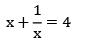x2 + 1 = 4x

x2 − 4x + 1 = 0

x = 3.73 or 0.26

*Answer can only contain numeric values
QUESTION: 14

Find the economic order quantity for a product of which the price breaks are as follows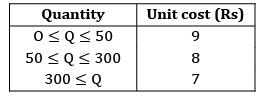The monthly demand for the product is 200 units. The cost of holding is 25% of the unit cost and the ordering cost is Rs. 20/order.

Solution: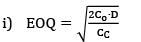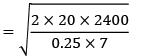[Annual demand = 12 × 200 = 2400] EOQ = 234.2

(Not falling in the range)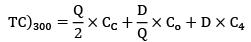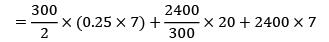= Rs. 17222.5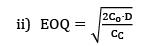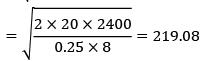(Falling under range)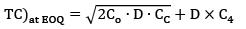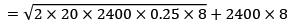= Rs. 19,638.17

Minimum cost is at 300 Quantity

QUESTION: 15

The monthly demand for an item is 150 units. The cost per unit is Rs. 30. Ordering cost is Rs. 50 per order and carrying cost is 25% of unit cost. The retailer offers 2% discount if 200 or more than 200 units are ordered at a time. The offer should be

Solution:

Given data,

D = 150 units/month

Annual demand D = 150 × 12 = 1800/year

Cu = Rs. 30/unit

Co = Rs. 50/order

CC = 25% × 30

= 0.25 × 30 = 7.5 Rs./unit/year

Q ≥ 200 then 2% discount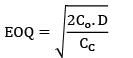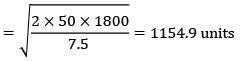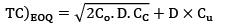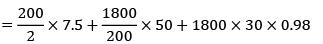= Rs. 54120

TC)200 < />

QUESTION: 16

A company has to manufacture 2,00,000 brackets in a year. It orders raw materials for the brackets in lots of 50,000 units from a vendor. It costs Rs. 40 to place an order, inventory carrying costs are 20% of the item cost/yr which is Rs. 0.2/unit. Calculate the variation of cost (in %) in their order quantity from optimal?

Ans. C

Solution:

Annual demand = 2, 00,000 units

Ordering cost = Rs. 40/order

Carrying cost = 20% of unit cost

Unit cost = Rs. 0.2/unit-year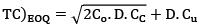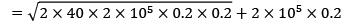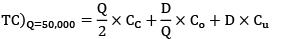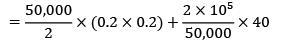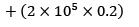= Rs. 41160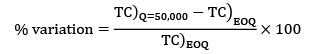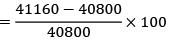= 0.88%

*Answer can only contain numeric values
QUESTION: 17

Consider an item with the following characteristics.

Annual demand = 400 /yr

Unit cost = Rs. 25

Inventory carrying cost = 20 % of unit cost

Cost of one procurement = Rs. 10

Shortage cost = Rs. 1.5/Unit-year

Based on the above data the time duration between two consecutive orders will be __________ months.

Solution:

Given data,

D = 400 units/year

Cu = Rs. 25

Cc = Rs. 0.2 × 25 = Rs. 125/unit-year

Cs = Rs. 1.5/unit-year

Co = Rs. 10/order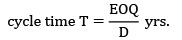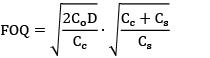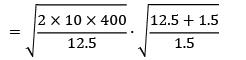= 77.28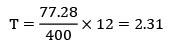QUESTION: 18

The total cost is represented with the following equation, TC = 10,500 +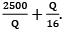Then TC)min is __________

Solution: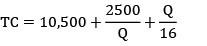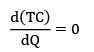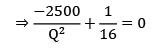Q = 2500 × 16

Q = 200 units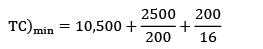TC)min = 10,500 + 12.5 + 12.5 = 10525